# Second-order mixed partial derivative

## Definition

### For a function of two variables

Suppose$f$ is a function of two variables which we denote$x$ and$y$. There are two possible second-order mixed partial derivative functions for$f$, namely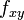$f_{xy}$ and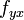$f_{yx}$. In most ordinary situations, these are equal by Clairaut's theorem on equality of mixed partials. Technically, however, they are defined somewhat differently.

Often the term mixed partial is used as shorthand for the second-order mixed partial derivative. However, mixed partial may also refer more generally to a higher partial derivative that involves differentiation with respect to multiple variables.

The following are all multiple equivalent notations and definitions of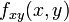$f_{xy}(x,y)$.

Name Notation Definition in terms of first-order partial derivatives
Subscript notation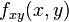$\! f_{xy}(x,y)$ Defined as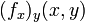$\! (f_x)_y(x,y)$. More explicitly:
Let$\! g(x,y) := f_x(x,y)$. Then,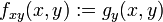$\! f_{xy}(x,y) := g_y(x,y)$.
Leibniz notation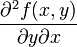$\frac{\partial^2f(x,y)}{\partial y \partial x}$ Defined as$\frac{\partial}{\partial y}\frac{\partial f(x,y)}{\partial x}$

Note that the order in which we write the$x$ and$y$ is different in the subscript and Leibniz notations because in the subscript notation, the differentiations are carried out from left to right (on subscripts) whereas in the Leibniz notation, the differentiations are carried out from right to left while simplifying.

The following are all multiple equivalent notations and definitions of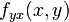$f_{yx}(x,y)$.

Name Notation Definition in terms of first-order partial derivatives
subscript notation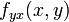$\! f_{yx}(x,y)$ Defined as$\! (f_y)_x(x,y)$. More explicitly:
Let$\! h(x,y) := f_y(x,y)$. Then,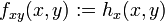$\! f_{xy}(x,y) := h_x(x,y)$.
Leibniz notation$\frac{\partial^2f(x,y)}{\partial x \partial y}$ Defined as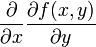$\frac{\partial}{\partial x}\frac{\partial f(x,y)}{\partial y}$

### For a function of many variables

For a function of more than two variables, we can define the second-order mixed partial derivative with respect to two of the variables (in a particular order) in the same manner as for a function of two variables, where we treat the remaining variables as constant. For instance, for a function$f$ of three variables$x,y,z$, we can consider the six mixed partials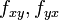$f_{xy}, f_{yx}$ (holding$z$ fixed),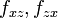$f_{xz}, f_{zx}$ (holding$y$ fixed),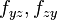$f_{yz},f_{zy}$ (holding$x$ fixed).

In general, for a function of$n$ variables, there are$n(n - 1)$ many second-order mixed partials that we can construct.

### Definition as a double limit at a point

We consider again the case of a function of two variables. In this case, the partial derivatives$f_{xy}$ and$f_{yx}$ at a point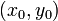$(x_0,y_0)$ can be expressed as double limits: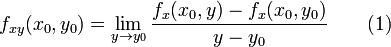$f_{xy}(x_0,y_0) = \lim_{y \to y_0} \frac{f_x(x_0,y) - f_x(x_0,y_0)}{y - y_0} \qquad \mbox{(1)}$

We now use that: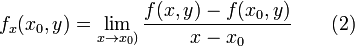$f_x(x_0,y) = \lim_{x \to x_0)} \frac{f(x,y) - f(x_0,y)}{x- x_0} \qquad \mbox{(2)}$

and:$f_x(x_0,y) = \lim_{x \to x_0)} \frac{f(x,y_0) - f(x_0,y_0)}{x- x_0} \qquad \mbox{(3)}$

Plugging (2) and (3) back into (1), we obtain that: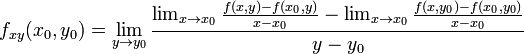$f_{xy}(x_0,y_0) = \lim_{y \to y_0} \frac{\lim_{x\to x_0} \frac{f(x,y) - f(x_0,y)}{x- x_0} - \lim_{x \to x_0} \frac{f(x,y_0) - f(x_0,y_0)}{x- x_0}}{y - y_0}$

A similar calculation yields that: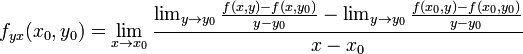$f_{yx}(x_0,y_0) = \lim_{x \to x_0} \frac{\lim_{y\to y_0} \frac{f(x,y) - f(x,y_0)}{y- y_0} - \lim_{y \to y_0} \frac{f(x_0,y) - f(x_0,y_0)}{y- y_0}}{x - x_0}$

As Clairaut's theorem on equality of mixed partials shows, we can, under reasonable assumptions of existence and continuity, show that these two second-order mixed partials are the same.

## Domain considerations

### For a function of two variables

Suppose$f$ is a function of two variables$x,y$. Consider a point$(x_0,y_0)$ in the domain of$f$. Suppose we are interested in determining whether$f_{xy}(x_0,y_0)$ exists. We can say the following:

• A necessary (though not sufficient) condition for$f_{xy}(x_0,y_0)$ to exist is that$f_x(x_0,y)$ exist for$y$ everywhere in an open interval containing$y_0$. In other words,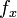$f_x$ exists at and near$y_0$ on the line$x =x_0$. Another way of saying this is that$f_x$ needs to exist not only at the point, but also if we perturb$y$ slightly.
• Building on this, a necessary (though not sufficient) condition for$f_{xy}(x_0,y_0)$ to exist is that$f$ should exist if we perturb$y$ a bit and then perturb$x$ a bit. It's tempting to believe that it is necessary that$f$ should be defined in an open neighborhood of the point$(x_0,y_0)$. However, this is not necessarily the case because it is not necessary that there exist a positive lower bound on the radius of the$x$-neighborhoods for definition of$f$ for$y$ close to$y_0$.

### For a function of more than two variables

Suppose$f$ is a function of variables$x_1,x_2,\dots,x_n$. Consider a point$(a_1,a_2,\dots,a_n)$ in the domain of$f$. Consider the mixed partial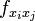$f_{x_ix_j}$ at$(a_1,a_2,\dots,a_n)$:

• A necessary (though not sufficient) condition for this second-order mixed partial to exist is that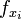$f_{x_i}$ be defined at points close to$(a_1,a_2,\dots,a_n)$ on the line where we fix all coordinates other than$x_j$ and allow$x_j$ to vary. Another way of saying this is that$f_{x_i}$ needs to exist not just at the point, but also if we perturb$x_j$ slightly.
• A necessary (though not sufficient) condition for this second-order mixed partial to exist is that$f$ be defined at points close to$(a_1,a_2,\dots,a_n)$ in the plane parallel to the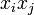$x_ix_j$-plane that passes through the point. Explicitly,$f$ needs to exist not just at the point, but at all points close to it obtained by perturbing$x_j$ slightly and then perturbing$x_i$ slightly.